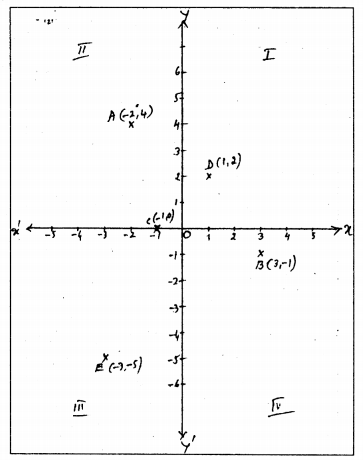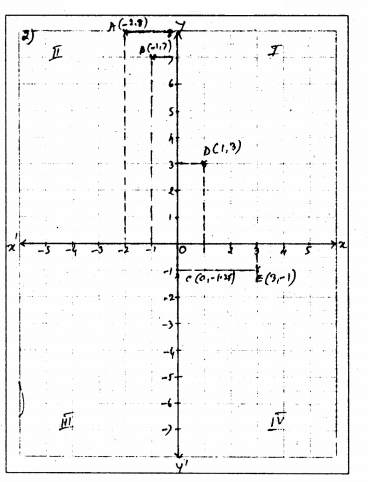# KSEEB Solutions for Class 9 Maths Chapter 9 Coordinate Geometry Ex 9.3

In this chapter, we provide KSEEB SSLC Class 9 Maths Chapter 9 Coordinate Geometry Ex 9.3 for English medium students, Which will very helpful for every student in their exams. Students can download the latest KSEEB SSLC Class 9 Maths Chapter 9 Coordinate Geometry Ex 9.3 pdf, free KSEEB SSLC Class 9 Maths Chapter 9 Coordinate Geometry Ex 9.3 pdf download. Now you will get step by step solution to each question.

## Karnataka Board Class 9 Maths Chapter 9 Coordinate Geometry Ex 9.3

Question 1.
In which quadrant or on which axis do each of the points (-2, 4), (3, -1), (-1. 0), (1, 2) and (-3, -5) lie? Verify your answer by locating them on the Cartesian plane.
Solution:C (-1, 0) Negative x-axis

Question 2.
Plot the points (x, y) given in the following table on the plane, choosing suitable units of distance on the axes.

Solution:
A                            B                              C                                D                                 EA (-2, 8)
B (-1, 7)
C (0,-1.25)
D (1, 3)
E (3, -1)

All Chapter KSEEB Solutions For Class 9 Maths

—————————————————————————–

All Subject KSEEB Solutions For Class 9

*************************************************

I think you got complete solutions for this chapter. If You have any queries regarding this chapter, please comment on the below section our subject teacher will answer you. We tried our best to give complete solutions so you got good marks in your exam.

If these solutions have helped you, you can also share kseebsolutionsfor.com to your friends.

Best of Luck!!